Home | | Physics 12th Std | Numerical Exercises Problems

# Numerical Exercises Problems

Physics : Atomic and Nuclear Physics: Book Back, Exercise, Example Numerical Question with Answers, Solution: Numerical Exercises Problems with Answers, Solution

Atomic and Nuclear Physics

Exercises

1. Consider two hydrogen atoms HA and HB in ground state. Assume that hydrogen atom HA is at rest and hydrogen atom HB is moving with a speed and make head-on collide on the stationary hydrogen atom HA. After the strike, both of them move together. What is minimum value of the kinetic energy of the moving hydrogen atom HB, such that any one of the hydrogen atoms reaches one of the excitation state.

[Ans: 20.4 eV]

Solution:2. In the Bohr atom model, the frequency of transitions is given by the following expression

Rc ( 1/n2 – 1/m2 ) , where n < m,

Consider the following transitions:Show that the frequency of these transitions obey sum rule (which is known as Ritz combination principle)

[Ans: v3→2  + v2→1 = v3→1]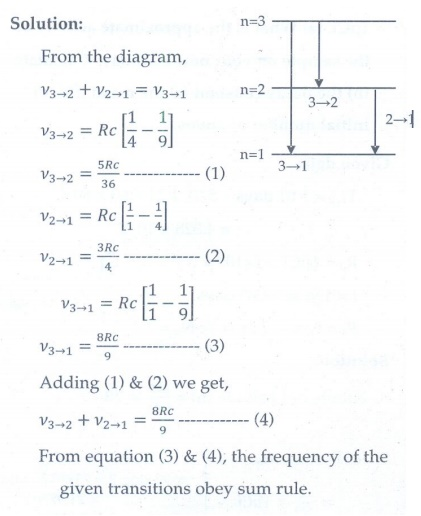3. (a) A hydrogen atom is excited by radiation of wavelength 97.5 nm. Find the principal quantum number of the excited state.

(b) Show that the total number of lines in emission spectrum is n(n-1) / 2 and  compute the total number of possible lines in emission spectrum.

[Ans: (a) n = 4 (b) 6 possible transitions]4. Calculate the radius of the earth if the density of the earth is equal to the density of the nucleus.[mass of earth 5.97 × 1024 kg ].

[Ans:180m]

Given data:

Mass of the earth = 5.97 x 1024 kg

Density of earth = Density of nucleus

= 2.3 x 1017 kg m-3

Solution: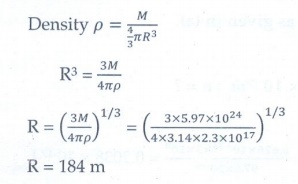5. Calculate the mass defect and the binding energy per nucleon of the 10847Ag nucleus. [atomic mass of Ag = 107.905949]

Ans: [∆= 0.990391and B.= 8.5MeV ]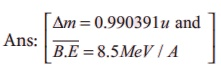Given data:

M = 107.905949 u

Z = 47      mH = 1.007825u

N = 61     mn =1.008665 u

Solution:6. Half lives of two radioactive elements A and B are 20 minutes and 40 minutes respectively. Initially, the samples have equal number of nuclei. Calculate the ratio of decayed numbers of A and B nuclei after 80 minutes.

[Ans: 5:4]

Given data:

T1/2 of A = 20 min

T1/2 of B = 40 min ; Total time = 80 min

NOA = NOB

NOA - NA  / NOB - NB = ?

Solutions:

Decayed number of nuclei = No – NThe ratio of decayed numbers of A and B is 5 : 4.

7. On your birthday, you measure the activity of the sample 210Bi which has a half-life of 5.01 days. The initial activity that you measure is 1μCi . (a) What is the approximate activity of the sample on your next birthday? Calculate (b) the decay constant (c) the mean life (d) initial number of atoms.

[Ans: (a) 10-22 μCi (b) 1.6 ×10-6 s-1 (c) 7.24days (d) 2.31×1010 ]

Given data:

T1/2 = 5.01 days = 5.01 × 24 × 60 × 60 s

= 4.328 × 105 s

R0 = 1 μCi = 1 × 10-6 × 3.7 × 1010 Bq

t = 1 year = 365 days

Rt = ? ; λ=?; τ = ?  ; N0 = ?

Solution: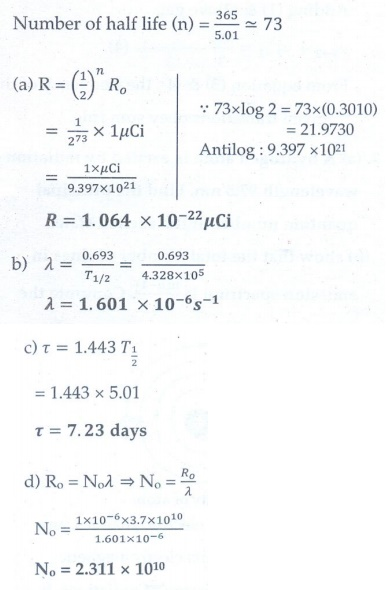8. Calculate the time required for 60% of a sample of radon undergo decay. Given T1/2 of radon =3.8 days

[Ans: 5.022 days]

Given data:

T1/2 = 3.8 days; λ= 0.693/3.8  per day

Amount of sample decayed = 60% of No

Amount of sample present (N) = 40% of No

No is initial amount of sample, t = ?

Solution:9. Assuming that energy released by the fission of a single 23592nucleus is 200MeV, calculate the number of fissions per second required to produce 1 watt power.

[Ans: 3.125 ×1010 ]

Given data:

Energy released during fission of single

23492U = 200MeV

Power = 1 watt = 1 J/s

No. of fissions per second required to produce

1W power = ?

Solution:

Energy produced = no. of fissions per second × energy released per fission

No.of fissions per second = energy produced / energy released per fission

= 1 / ( 200 ×106 ×1.6 ×10-19)

= 1/ (320 × 10-13)

= 1013/320 = 3.125 ×1010

No. of fissions per second = 3.125 × 1010

10. Show that the mass of radium ( 22688Ra ) with an activity of 1 curie is almost a gram. Given T1/2 =1600 years.

Given: T1/2 =1600 years (PTA-6)

Given data:

Ro = 1Ci= 3.7 × 1010  Bq

T1/2    = 1600 years

Mass of radium with an activity of 1 Ci= ?

Solution:The mass of radium with an activity of 1 Ci is almost a gram.

11. Characol pieces of tree is found from an archeological site. The carbon-14 content of this characol is only 17.5% that of equivalent sample of carbon from a living tree. What is the age of tree?

[Ans: 1.44 × 104 yr]

Given data:

The amount of 14C present (N) = 17.5% of No

T1/2 = 5730 years

Age of the tree (t) = ?

Solution: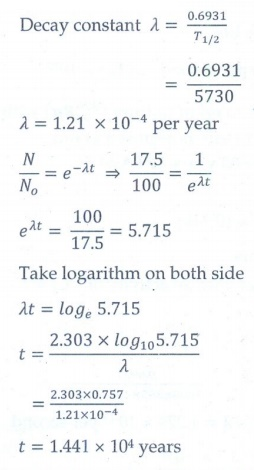Tags : with Answers, Solution | Atomic and Nuclear Physics | Physics , 12th Physics : UNIT 9 : Atomic and Nuclear Physics
Study Material, Lecturing Notes, Assignment, Reference, Wiki description explanation, brief detail
12th Physics : UNIT 9 : Atomic and Nuclear Physics : Numerical Exercises Problems | with Answers, Solution | Atomic and Nuclear Physics | Physics